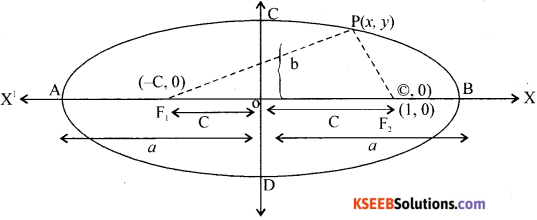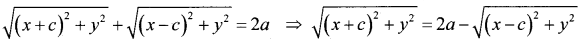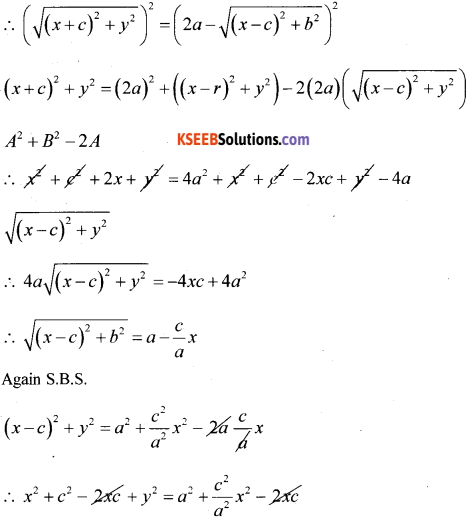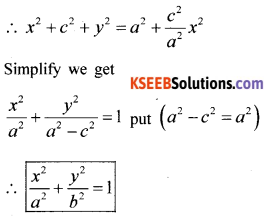# 1st PUC Maths Model Question Paper 2 with Answers

Students can Download 1st PUC Maths Model Question Paper 2 with Answers, Karnataka 1st PUC Maths Model Question Paper with Answers helps you to revise the complete Karnataka State Board Syllabus and score more marks in your examinations.

## Karnataka 1st PUC Maths Model Question Paper 2 with Answers

Time: 3 Hrs 15 Min
Max. Marks: 100

Instructions:

1. The question paper has five parts namely A, B, C, D and E. Answer all the parts.
2. Part-A carries 10 marks. Part-B carries 20 marks. Part-C carries 30 marks. Part-D carries 20 marks, Part-E carries 10 marks.

Part – A

Answer ALL the questions. Each question carries one mark. (10 × 1 = 10)

Question 1.
If n(A) = 3, then find n[P(A)].
n(A) = 3 then n[p(A)] = 23 = 8 elements.

Question 2.
If (x – 2, y + 3) = (5, -6), then find the values x and y.
x – 2 = 5 ∴ x=7
y + 3 = -6 ∴ y=-9.

Question 3.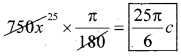Question 4.
Find the conjugate of i(2 + i).
Conjugate i(2 + i) = 2i + i2 = -1 + 2i
∴ conjugate Z̄ = -1 -2i.Question 5.
If nC5 = nC10, then find n
n = 5 + 10 = 15

Question 6.
Find the 5th term of the GP. $$1, \frac{1}{5}, \frac{1}{25},$$
a5 = (1)$$\left(\frac{1}{5}\right)^{5-1}$$ = $$\left(\frac{1}{5}\right)^{4}=\left[\frac{1}{625}\right]$$

Question 7.
Find the slope of the straight line 3x – 4y + 6 = 0
Stage = m = $$-\frac{(3)}{-4}=\left[\frac{3}{4}\right]$$

Question 8.
Evaluate $$\lim _{x \rightarrow 4} \frac{x^{3}-64}{x-4}$$
= n : an-1 = 3 (4)3-1 = 3(4)2 = 48

Question 9.
Write the negation of “Every natural number is greater than 0″.
“Every natural number is not greated than 0.”

Question 10.
Define “sample space”.
Sample space: Set of all possible outcome of an event is called a sample space.

Part – B

Answer any TEN Questions. (10 × 2 = 20)

Question 11.
If U = {x : x ≤ 6 and x ∈ N}, A = {3,5}, B = {2,5,6} then find (A ∪ B)’.
A ∪ B = {3,5} ∪ [2;5,6] = [2,3,5,6]
∴ (A ∪ B)’ = {1,4}.Question 12.
If X and Y are two sets such that n(X ∩ Y) = 10, n(X) = 38, n(Y) = 25 then find – n(X ∪ Y).
If n(X ∪ Y) = n(X) + n(Y) – n(X ∩ Y) = 38 + 25 – 10 = 53

Question 13.
Finid the domain and range of the real function f(x) = $$\sqrt{9-x^{2}}$$
Domain of f(x) = {x : x ∈ 2, -3 ≤ x ≤ 3}, Range of f(x)=[x : x ∈ R]

Question 14.
If in two circles, arcs of the same length subtend angles 60° and 75o at the centre, then find the ratio of their radii.
S1, = 5, S2 = 5, θ1 = 60° = $$\frac{\pi^{c}}{3}$$ r1 : r2 = ?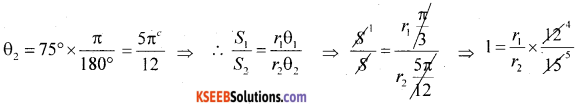∴ r1 : r2 = 5 : 4

Question 15.
Find the value of sin 75°
sin 75° = sin ( 45° +30°)
[sin (x + y)= sin x cos y + cosxsin y] = sin 45° . cos 30° + cos 45°. sin 30°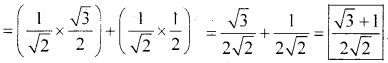Question 16.
Express the following in a + ib form $$\left[\left(\frac{1}{3}+i \frac{7}{3}\right)+\left(4+i \frac{1}{3}\right)\right]-\left(-\frac{4}{3}+i\right)$$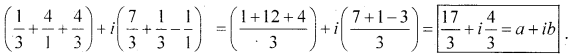Question 17.
Solve thr inequality 3(1 – x) <2 (x + 4) and represent it on number line
3(1 – x) <2 (x + 4) ⇒ ∴ 3 – 3x < 2x + 8
⇒ ∴ 3 – 8 < 2x + 3x
-5 < 5x ⇒ -1 < x or x > -1.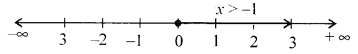Question 18.
Find the acute angle between the straight lines 5x + 6y – 1 = 0 and x – 11y + 8 = 0.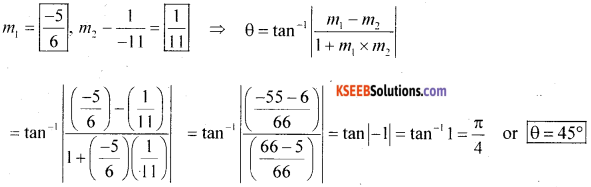Question 19.
Find the distance between the parallel lines 3x – 4y + 7 = 0 and 3x – 4y + 5 = 0.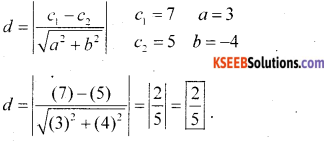Question 20.
Tind the ratio in which the YZ – plane divides the line segment formed by joining the points (-2,6,7) and (2,-5,6) internally.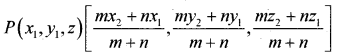Let the ratio be m : n = k : 1 (Assume)
(x1, y1, z1) = (-2,6,7) ⇒ (x2, y2,z2) = (2,-5,6)
yz plane divides the x coordinate is = 0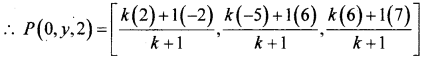∴ 0 = $$\frac{2 k-2}{k+1}$$ ⇒ ∴ 0 = 2k – 2 ∴ k = 1 ∴ ratio is 1 : 1

Question 21.
Evaluate $$\lim _{x \rightarrow 0} \frac{1-\cos x}{x}$$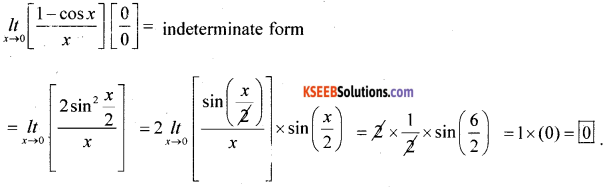Question 22.
Write the converse and contrapositive of “If two lines are parallel then they do not intereset in the same plane.”
Converse: (q → p) If two line donot interest in the same plane then they are parallel,
Contrapositive (~q → -p): If two line intersect in the same plane then they are not paralle..

Question 23.
The coefficient of variation of a distribution is 60 and the standard deviatio is 21.What is the arithmetic mean of the distribution?
C.V.= 60, σ = 21, X̄ = ? .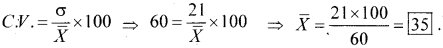Question 24.
A letter is choosen at random from the word “ASSASSINATION”. Find the probability that the letter is (i) a vowel (ii) a consonant.
Total letters = 13
Vowels = AAAIIO = 6
Consonant = SSSSNNT = 7
‘A’ repeas = 3 times. I repeats = 2
‘S’ repeats. 4 times. N repeats = 2
Probability of choosen a letter it a vowel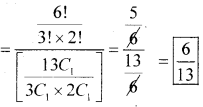Probability of choosen a consonant = $$\frac{7 C_{1}}{13 C_{1}}=\left[\frac{7}{13}\right]$$

Part – C

Answer any TEN of the following questions. Each question carries THREE marks. (10 × 3 = 30)

Question 25.
In a class of 35 students, 24 like to play cricket and 16 like to play football. Also each student likes to play atleast one of the two grams. How many students like to play both cricket and football?
Cricket = C, Football = F, n(C ∪ F) = 35, n(C ∩ F) = ?
n(C) = 24 2(F) = 16
n(C ∩ F) = n(C) + n(F) – n(C ∪ F) = 24 + 16 – 25 = 40 – 35 = 5

Question 26.
Let f = {1,1),(2,3)(0,-1),(-1-3}} be a linear function from Z into Z. Find f (x).
Let the function be f (x) = ax + b
f (1) = a(1) + b = a + b = 1 ⇒ f(2) = a(2) + b = 2a + b = 2
f(0) = a(0) + b = [b = -1] …..(1)
f(-1) = a(-1) + b = -3
∴ b – a = -3 … (2)

(i) in (2) (-1) -a = -3
∴ -1 + 3 = a ⇒ ∴ a = 2 ⇒ ∴ f(x) = 2x – 1.Question 27.
Find the general solution of the euation cos 4x = cos2x.
cos 4x = cos 2x , cos 4x – cos 2x = 0
By transformation formula cosC – cos D = -2 sin $$\left(\frac{C+D}{2}\right)$$ sin2 (-1)
-2 sin$$\left(\frac{6 x}{2}\right)$$ × sin $$\left(\frac{2 x}{2}\right)$$ = 0
∴ 2 sin 3x × sin x = 0 or sin 3x = 0 or sin x = 0
2 = 0°
∴ General Solution for sin x is
x = nπ + (-1)nα ⇒ 3x = nπ + 0 ∴[x = $$\frac{n \pi}{3}$$ or nπ]
or
cos 4x = cos 2x ⇒ assume α = 2x
∴ General solution
x = 2nπ ± 2 ⇒ 4x = 2nπ ± 2x ⇒ ∴ 2x = 2nπ and 6x = 2nπ
x = nπ, x = $$\frac{n \pi}{3}$$

Question 28.
Solve the equation √2x2 + x + √2 = 0. .
a = √2, b = 1, c = √2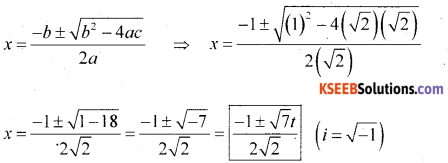Question 29.
Express the complex number -1 + i√3 in polar form.
-1 + i√3 = r(cos θ + isin θ) polar form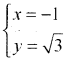∴ r = $$\sqrt{x^{2}+y^{2}}=\sqrt{1+3}=\sqrt{4}=2$$
θ = tan-1($$\left(\frac{y}{x}\right)$$) = tan-1√3 – 1 = -(π – Standard angle) = $$-\left(\pi-\frac{\pi}{3}\right)=\frac{(-2 \pi)}{3}$$
∴ -1 + i√3 = 2cis$$\left(\frac{-2 \pi}{3}\right)$$

Question 30.
How much words.with or without meaning can be made from the letters of the word “MONDAY” assuming that no letter is repeated if
(i) Four letters are used at a time
(ii) All letters are used at a time
(iii) All letters are used but first letter is vowel?
Monday: Given 6 letters
∴ number of arrangement = 6P6 = 6!
(i) Taken 4 letters at a time
= 6P4 = $$\frac{6 !}{2 !}$$ = 6 × 5 × 4 × 3 = 360 ways

(ii) all letters at a limt = 6P6 = 6! = 720 ways.

240 ways.Question 31.
Find the middle terms in the expansion of $$\left(\frac{n+1}{2}\right)$$ and $$\left(\frac{n+1}{2}\right)$$ + 1
x = 3 , a = $$-\frac{x^{3}}{6}$$, n = 9, T5 = ?, r = 4
Formula Tr+1 = nCr xn-r, ar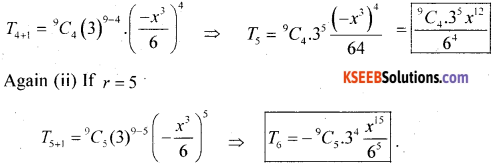Question 32.
Insert five numbers between 8 and 26 such that the resulting sequence is an arithmetic progression.
8A1, A2, A3, A4, A5,26
a = 8, n = 7, an = 26, d = ?
an = a + (n-1)d ⇒ 26 = 8 + (7 – 1)d = 26 – 8 = 6d
18 = 6d ∴ d=3
Common difference
∴ A1 = a + d = 8+3 =11, A2 =11 + 3 = 14, A3 = 17, A4 = 20, A5 = 23 are the 5 AM’s.

Question 33.
The sum of first three terms of a geometric progression is $$\frac { 39 }{ 10 }$$ and their product is 1. Find the common ratio and the terms.
Let the 3 term in GP are $$\frac { a }{ r }$$ a and ar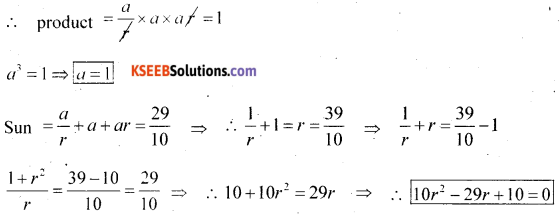Qudratic equation
∴ 10r2 – 25r – 4r + 10 ⇒ 50(2r – 5) -2 (2r – 5) = 0
(2r – 5) = 0 or 5r – 2 = 0 ⇒ ∴ r = $$\frac{5}{2}$$ or r = $$\frac{2}{5}$$
∴ the three terms are
at a = 1, r = $$\frac{5}{2}$$
I term $$\frac{a}{r}=\frac{1}{\frac{5}{2}}=\frac{2}{5}$$
II term a = 1
III term ar = $$\frac{5}{2}$$

at a = 1, r = $$\frac{2}{5}$$
I term ar = $$\frac{5}{2}$$
II term a = 1
III term = $$\frac{2}{5}$$

Question 34.
Find the equation of the circle with radius 5, whose centre lies on x-axis and passes through the point (2, 3).
r = 5 .
Centre lies on X – axis ⇒ pt(2,3) = (x, y)
Let the circle equation be x2 + y2 + 2fx + 2gx + C =0 … (1)
∵ centre lies on x-axis ∴ f = 0
∴ (1) becomes
x2 + y2 +2 gx + c = 0) at (2,3)
4 + 9 + 2g(2) + c = 0 ∴ 48 + c = -13 …(2)
∴ $$\sqrt{g^{2}+f^{2}-c}=5 \mathrm{SBS}$$
Put f = 0. :g2 – c = 25 …(3)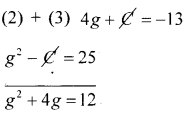∴ g2 + 4g – 12 = 0 ⇒ g2 + 5g – 2y = 12 = 0 ⇒ g(g – 16) – 2(g + 6)=0
∴ g = -6 or g = -2
Substitute in (2) 4g + C = -13
at g = -6 4(-6) + C = -13 ⇒ C = -13 + 24 = 11
at g = -2 4(-2) + 1 = -13 ⇒ C =-13 + 8 = -5
∴ at g = -6, C = 11, f = 0 (1) becomes
x2 + y2 – 12x + 11 = 0
at g = -2, c = -5, f = 0
x2 + y2 – 4x – 5 = 0

Question 35.
Find the derivative of sin x with respect to x fron first principles.
y = sin x as x → x + Δx, y + Δy
∴ (y + Δy) = sin(x + Δx)
Δy = sin(x + Δx) – y
Δy = sin(x + Δx) – sin x
÷ Δx and apply lt Δx → 0 on both side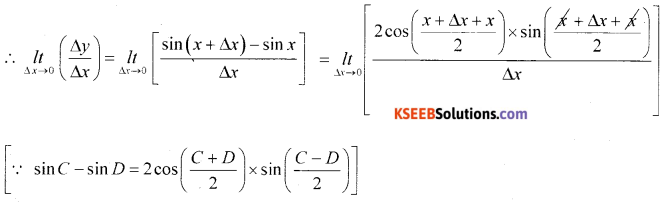Question 36.
Verify by the method of contradiction that “√7 is irrational.”
Prove that √7 is irrational
Assume √7. is an rational :: √7 = $$\frac{p}{q}$$
Squaring on b.s.
∴ 7 = $$\frac{p^{2}}{q^{2}}$$
∴ 7q2 = p2 i.e., p in a multiple of again put p = 7k (k+1) :
∴ 7q2 = (7k)2 ⇒ 7q2 = 49k2 ⇒ q2 = 7k2
⇒ q is also a multiple of q ⇒ ∴ both “p’ and ‘q’ are the factor of 7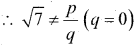∴ our assumption that √7 is an partial is wrong. ∴ √7 is an irrational.Question 37.
A committee of two pers.ns ¡s selected from 2 men and 2 women. What is the probability that the committee will have
(i) no man (ii) one man.
Given 2 mens × 2 women → select 2 person.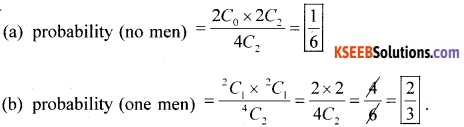Question 38.
If E and F are two events such that P(E) = $$\frac { 1 }{ 4 }$$ and P(F) = $$\frac { 1 }{ 2 }$$ and P(E and F) = $$\frac { 1 }{ 8 }$$ then find p(not E and not F)
P(E) = $$\frac { 1 }{ 4 }$$, P(F) = $$\frac { 1 }{ 2 }$$, and p(E ∪ F) = $$\frac { 1 }{ 8 }$$ P(A ∩ F) = 1
p(E ∩ F) = p(F) + p(F) – p(E ∪ F) = $$\frac{1}{4}+\frac{1}{2}-\frac{1}{8}=\frac{2+4-1}{8}=\frac{5}{8}$$

Part – D

Answer any SIX Questions. (6 × 5 = 30)

Question 39.
Define signum function Draw its garph. Write its domain, co – domain and range
Signum function: The function f:R → R defined by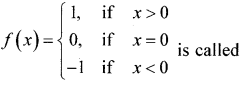a signum function: The domain of the signum function is R (Real nos) and the bugs and the signum function is the set {-1,0,1}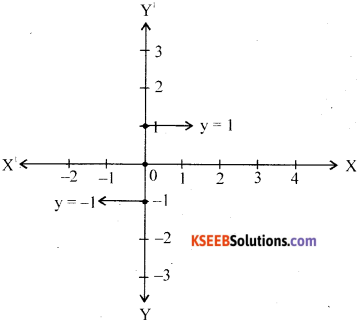Question 40.
Prove that $$\frac{\sin 5 x-2 \sin 3 x+\sin x}{\cos 5 x-\cos x}=\tan x$$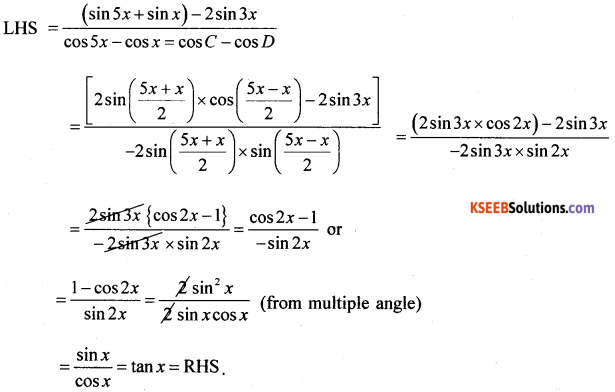Question 41.
Prove that 1.2 + 2.3 + 3.4 + ……….. +n(n – 1) = $$\frac{n(n+1)(n+2)}{3}$$ ∀n ∈ N, by using principal of mathematical induction.
at n = 1
LHS = RHS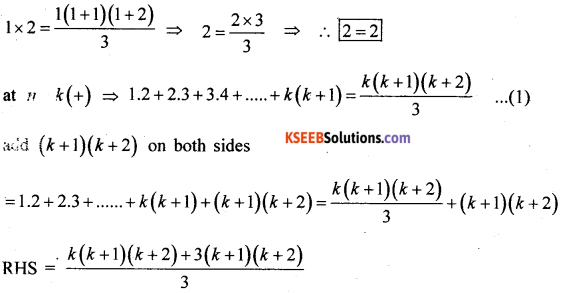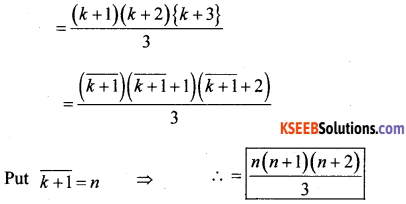Hence the given statement is true for n =1,2,…..k, k +1…. i.e., for all (+) integral value of n.

Question 42.
Solve the system of linear inequalities: 4x + 3y ≤ 60, y ≥ 2x, x ≥ 3, y ≥ 0 graphically.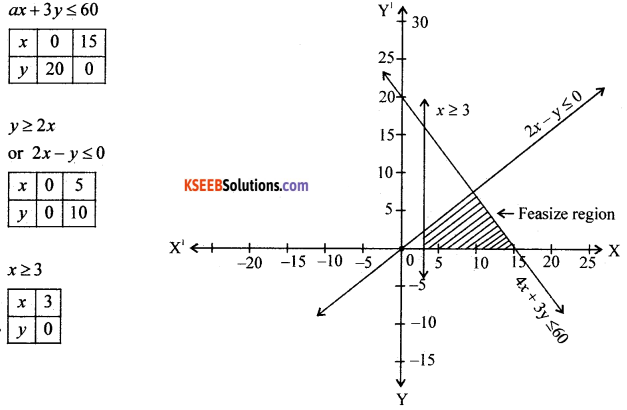Question 43.
What is the number of ways of choosing 4 cards from a pack In how many of these (i) four cards are of the same suit? (ii) four card belong to four different suits?
Given 52 cards → select 4 cards.
(i) 4 cards are of same smit.
∴ total number of selection $$=\left[\frac{13 C_{4}+13 C_{4}+13 C_{4}+13 C_{4}}{4}\right]$$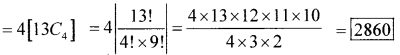(ii) 4 cards are of different suits
Total number of selection = 13C1 × 13C1 × 13C1 × 13C1 = (134)4 = (13)4 = 28.561

Question 44.
Prove binomial theorem for any positive integer n.
Prove binomial theorem for any positive integral.
Statement: (a + b)n =nC0an + nc1an-1 b + nC2 an-2 b2 + nC3 an-3 b3 + …….. + nCn-1 a.bn-1 + nCnbn
Proof: By aplying principle of mathemaical induction
Let P(n) : (a + b)n = nC0an + nc1an-1b1 + ……. + nC nbn
For n = 1 p(1) = (a + b)1 = 1C0a1 + 1C1a1-1b1
[(a + b) = (a – b)] is true
For n = K(f). p(k) = (a + b)k = kC0ak + kC1ak-1b +….+ KCkbk …..(l)
is true for n = k
We shall prove that p(k + 1) is also true i.e..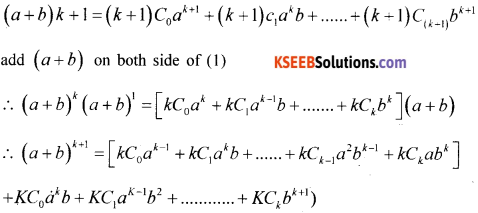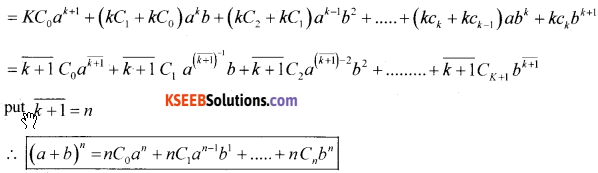Thus it has been proved that R(k + 1) is tense for p(K) is true and it is that for all positive integer n.

Question 45.
Derive the equation of straight line in the normal form x,cos θ + y,sinω = P, where Po is the length of normal to the line from the origin and ‘ω’ is inclination of normal with positive x-axis.
Derive the eqn. of straight line in the following
x cos ω + y sin ω = p (normal form)
consider a line cutting x-axis at A and y-axis at B.
Let the x-intercept = OA = a y-intercept OB = b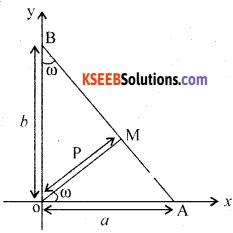Drawn OM ⊥ to AB from 0
OM is called the length of the normal = P. It makes an angle of the norma = P. It makes an angle w (omega) w.r.t. X-axis.
In the angle AOM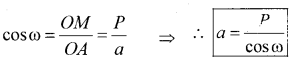In the angle ROM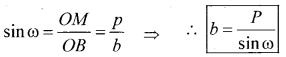Equation of the line in intercept is given by $$\frac{x}{a}+\frac{y}{b}=1$$ substitute ‘a’ and ‘b’ then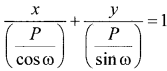∴ [xcosα w + ysin α = p] in the equation of line AB is normal form.Question 46.
Derive the expression for the co-ordinates of a point that divides the line joining points A(x1,y1,z1) and B(x2, y2,z2) internally in the ratio m:n, also find the midpoint of line AB, where A = (2,8,1) and B = (2,-2,5).
Proof: Let p(x1, y1, z1) and Q(x2, y2, z2) be the given points
Let R(x, y, z) divide PQ internally in the ratio m : n
Draw PL, QM, RN perpendicular to xy-plane.
∴ PL ∥ RN ∥ QM
∴ PL, RN, QM lie in one plane
So that the points L, N, M lie in a straight line which is the intersection of the plane and XY plane.
Through the point R draw a line AB It to the line LM. The line AB intersect the line LP externally at A and the line MQ at B.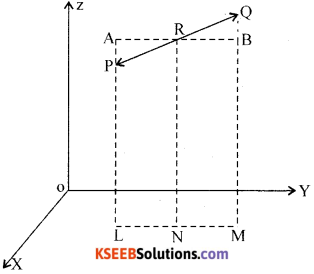Triangle APR and LIQR are similar.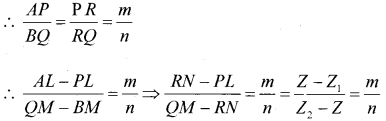∴ n(z – z1) = m(z2 – z)
∴ nz = nz1 = mz2 – m2
∴ (nz + mz) = (mz2 + nz1)
∴ z(m + n) = mz2 + nz1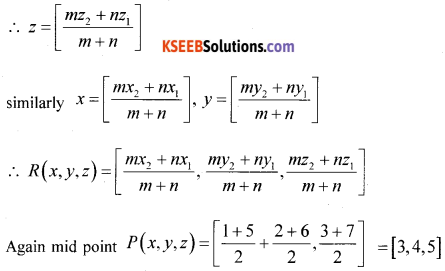Mid point of AB = $$=\frac{2+2}{2}, \frac{F-2}{2}, \frac{1+5}{2}=(2,3,3)$$

Question 47.
Prove that $$\lim _{x \rightarrow 0} \frac{\sin x}{x}=1$$ Where “x” being measured in radians.
$$\lim _{\theta \rightarrow 0} \frac{\sin \theta}{\theta}=1$$
Proof: Consider a circle with centre ‘O’ and radius ‘r’. Mark two point A and B on the circumference of the circle so that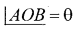At ‘A’ draw a tangent to the circle produce OB to cut the tangent at C. Joint AB.
Draw BM ⊥ OA,
Here OA = OB = r
From the figure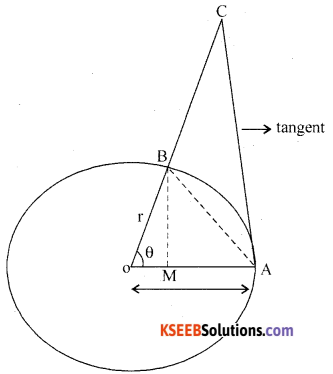Area of triangle OAB < area of the sector AOB < area of triangle OAC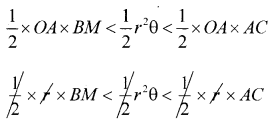∴ BM < rθ < AC … (1)
In triangle OBM : sinθ = $$\frac{B M}{O B}$$
∴ BM = OB sinθ = rsinθ
In triangle OAC: tan θ = $$\frac{AC}{O A}$$ ∴ AC = OA tan θ = r tan θ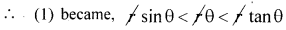sin θ < 0< tan θ
÷ sin θ
$$1<\frac{\theta}{\sin \theta}<\frac{1}{\cos \theta}$$
apply lt θ → 0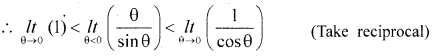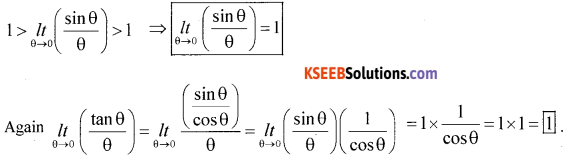Question 48.
Find the mean deviation about mean for the following data: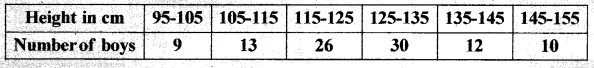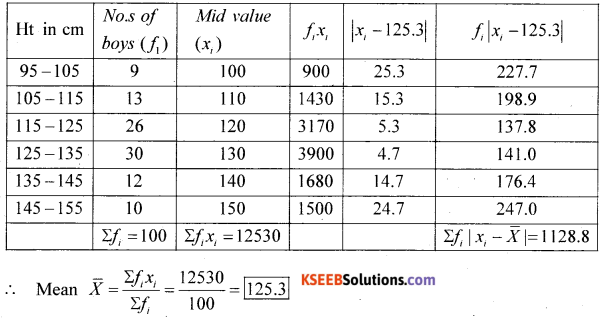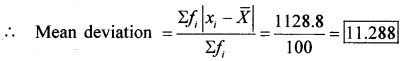Part – E

Answer any ONE question. (1 × 10 = 10)

Question 49.
(a) Defind Hyperbola as a set of points and derive the equation of hyperbola in standard form $$\frac{x^{2}}{a^{2}}-\frac{y^{2}}{b^{2}}=1$$
Definition hyperbola: A Hyperbola is the act of all points in a plane the difference of whose distances.
From two fixed points in the plane is constant.
The two fixed points are called Focii and the mid point ‘O’ is called the centre of the hyperbola.
Distance between two focii F1 and F2 = 2c
Distance between two velocities = AB = 2a
(length of transverse axis) and b = $$\sqrt{c^{2}-a^{2}}$$
∴ 2b = length of conjugate axis and $$e=\frac{c}{a}$$ is called the eccentricity of the hyperbola.
Derivation : Equation of hypergola in standard form $$\frac{x^{2}}{a^{2}}-\frac{y^{2}}{b^{2}}=1$$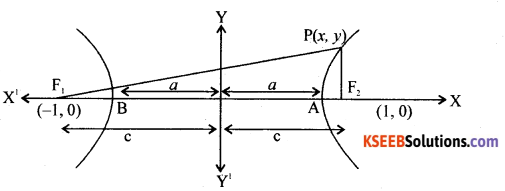From the definition PF1 – PF2 = 2a
Using the distane formula
$$\sqrt{(x+1)^{2}+y^{2}}-\sqrt{(x-c)^{2}+y^{2}}=2 a$$
∴ $$\sqrt{(x+c)^{2}+y^{2}}-\sqrt{(x-c)^{2}+y^{2}}=2 a$$
Squaring on both side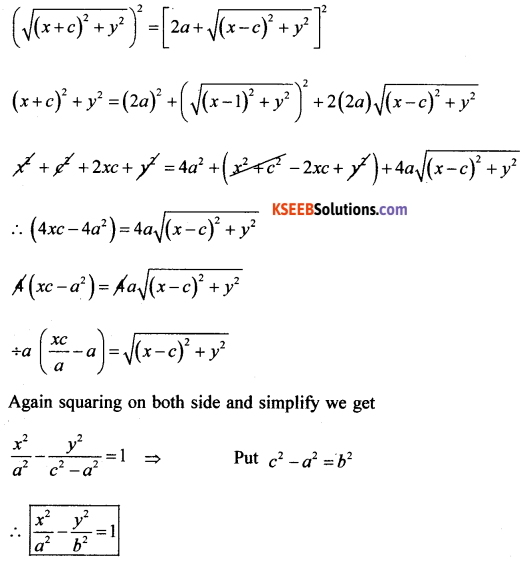(b) Find the deviation of $$\frac{x^{2}-\cos x}{\sin x}$$ with repect to x.
y = $$\left[\frac{x^{5}-\cos x}{\sin x}\right]$$ by using quotient rule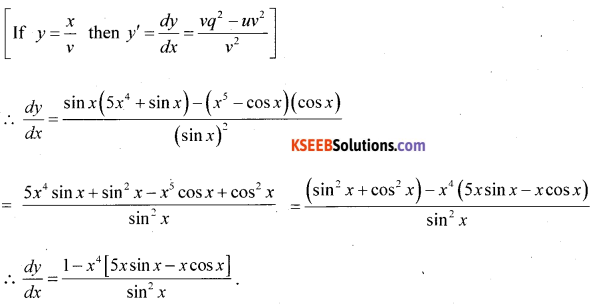Question 50.
(a) Prove geometrically that cos(x + y) = cos x, cos y – sin x.sin y.
Prove that cos(x + y) = cos x cos y.sin x sin y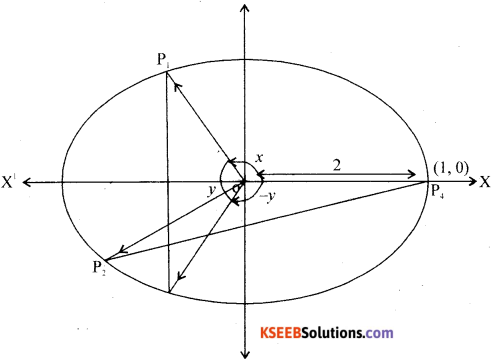w
Proof Consider a unit circle (radius = 1 unit) with centre is (0, 0).
Consider 4 point P1, P2, P3 and P4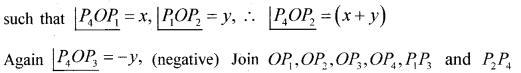The co-ordinate of P1, P2, P3 and P4 are given by
P1 = (cosx,sinx) P2 = [cos(x + y). sìn(x + y)]
P3 = [cos(-y) sin(-y)] P4 = [1,0]
From the figure OP1OP3 is congruent to ∆P2OP4
∴ From distance formula
P1p3 = P2P4 …… (1)
Takè the distance (P1P3)2 = [cosx – cos(-y)]2 + [sin x – sin (-y)]2
= (cosx – cosy)2 + (sin x + sin y)2
= cos2x + cos2y = cosxcosy + sin2x + sin2y + 2sin cosy = 1 + 1 + 2(cosxcosy – sinxsiny)
(p1, P3)2 = 2 – 2 cos (x + y)
Again (P2P4)2 = [1(a-b)2-cos(x+y)]2 + |q – sin (x + y)|2
= 1 + cos2(x + y) -2cos(x + y) + sin2(x + y) = 1 + 1 – 2cos(x + y) = 2 – 2cos(x + y)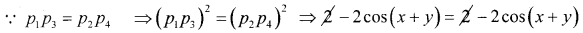⇒ LHS = RHS
∴ [cos(x + y) = cosx cosy – sin x sin y]

(ii) Show that cos 2x = cos2x – sin x2x
Take cos(x + y) = cos xcos y – sin xsin y
Put y = x
∴ cos(x + x) = cosx cosx – sinx sinx
cos2x = cos2 x – sin2x(b) Find the sum to n terms of the